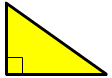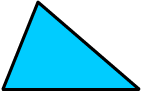Skill 23F Triangles: Right, Obtuse and Acute While skill 23E categorized triangles according to the length of their sides, this skill categorizes triangles according to the measurement of the angles in a triangle.  For example, since the yellow triangle below has one right angle, it is called a right triangle (a right angle has a measurement of 90 degrees and is designated in textbooks with the small square in the corner).   If the triangle has one angle more than 90 degrees (as in the second purple triangle), it is called an obtuse triangle.  If all three angles in the triangle are less than 90 degrees (as in the third blue triangle), it is called an acute triangle (maybe because they thought it was prettier than the others)...  To take this one step further, you could say triangle number 2 is a scalene obtuse triangle since its sides are all different lengths (which is what designates a scalene triangle) and it has one angle more than 90 degrees.  So, to give you a small trick to put in your pocket, use the 1-1-3 rule for categorizing triangles according to their angles.  1 right angle = right triangle 1 angle more than 90 degrees = obtuse triangle 3 angles less than 90 degrees = acute angle Triangles can also be given names depending on the type of angles in the triangle.  Tell whether each triangle is a right triangle, obtuse triangle or acute triangle. 1. 2. 3.? ? ? right triangleobtuse triangle acute triangle right triangleobtuse triangle acute triangle right triangleobtuse triangle acute triangle